Project 1a - Subdivide, Render, Animate
Goal: The goal of this phase of the project was to develop or adapt a 2-dimensional polygon editor and the process for turning that polygon into a subdivided curve. The curve was to be drawn in the shape of a road signifying a race track. Two dots representing cars would move along this road in separate animation modes. The first mode, parametric speed, would move the car a fixed number of steps for each vertex-vertex pair. The second mode, constant speed, would move the car a fixed step distance for the entire length of the race track.

System: My project was written on Windows XP using Processing (http://processing.org). The Java wrapper used by Processing should make it cross-platform compatible.

Implementation: I decided to implement this part of the project in Processing for several reasons. The first is that there was already a polygon editor and subdivider I could adapt (written by Professor Rossignac) written in Processing. The second is that Processing has a simple interface and has a small learning curve, which is necessary since I have no previous OpenGL or other C graphic library experience. I made several modifications and enhancements to the original code. First of all I change the curve to look like a race track. I also did some optimization by only recalculating the curve only when a modification to a control point was made. I then added in methods to draw the cars and calculate their next position using both animation mode types. My project was written on Windows XP but the Java wrapper used by Processing should make it cross-platform compatible.

Applet:
To view this content, you need to install Java from java.com

Source Code: P1a.pde

Demo Video: p1a-demo-divx.avi (3 MB, DivX Required)

Demo Pictures:
(click to enlarge)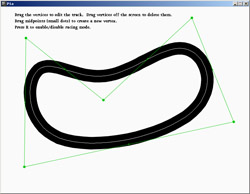This is a picture of what the applet looks like when first run.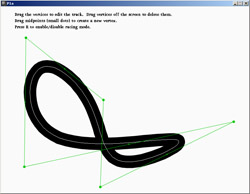This is after a track edit -- the upper right vertex / control point has been dragged to the bottom.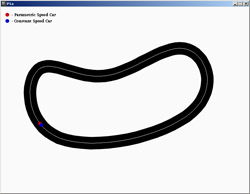Soon after the race starts the cars are nearly tied.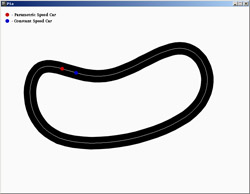After the first bend the constant speed car is winning because it takes larger steps than the parametric car.

Subdivision Scheme: I choose to utilize the Split and Tweak method to create a Uniform Cubic B-spline curve. The reason for this is that the Split and Tweak Cubic B-spline is C2 and rounds corners sufficiently to allow smooth travel by a moving vehicle. The only disadvantage to this method is that it makes the curve relatively smaller compared to the original editing polygon. The basic algorithm is as follow: For each vertex compute a displacement vector V.d=(VV.l+VV.r)/8. Split each edge to create a new vertex. Finally move each vertex to V + V.d.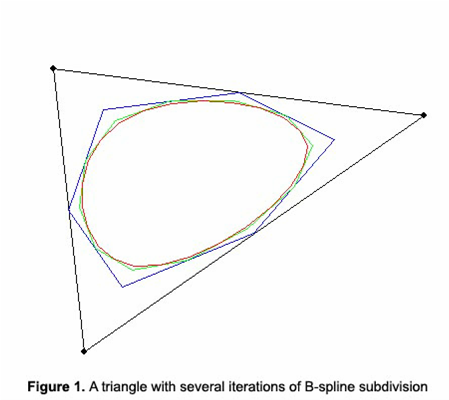(Jarek Rossignac - Subdivision curves and surfaces, slide 50)

Animation Modes: The first animation mode I implemented was the parametric speed mode. This was comparatively easier than the constant speed mode for reasons I will explain. First of all, each car has several global variables that are updated one step by the moveCar() method. Each car has an x and y position in the window that is updated at the end of each mode's computation. Car 1 (the parametric car) has a global vertex_id variable and step_number variable. At each call to moveCar(), the car is moved forward by one step. At first the number of steps per vertex was a fixed number. I later changed this to approximate a pixels per second velocity approach. I think this will come in handy later when we have to deal with acceleration. The next step for Car 1 x coordinate is calculated using car1_posx = qx[car1_vert] + (car1_stepnum * deltax) where deltax = (qx[nextVertex(car1_vert)] - qx[car1_vert]) / steps_per_side, and equivalently for the y coordinate. After each iteration the step number is incremented, or if the car is at the last step the vertex number is incremented. It is worth noting that the pixels / second calculation is only and approximation for parametric speed mode since an average is taken of the length of all edges, and it could be that there are many small edges leading to a slower than expected speed and vice versa. Car 2, which moves in constant speed mode, proved to be more of a challenge to implement. When car 2 starts at a vertex, it moves a constant fixed distance towards the next vertex. If there is not enough room it subtracts the length already moved along the first edge and proceeds to the next edge. At first I could do get this method to work correctly until I realized that some "next edges" were still too small to accommodate the car. This required me to code a loop looking for the next edge that is "big enough". Each method uses one pixel-side lengths to make their calculations so both should be as numerically accurate as possible.